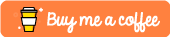What Is Bubble Sort?

Bubble sort is a sorting algorithm that sequentially goes through an array n times. Each time the algorithm runs through our array, it looks at the first element and then the second element, if the first element is larger than the second element then it swaps them, it then proceeds through the entire list performing this action.

Time Complexity

The time complexity for this algorithm is O(n^2) where n is the number of items being sorted. An example of a worst case scenario would be:

[9,8,7,6,5,4,3,2,1,0]

In this case the entire array is backwards and as a result the bubble sorting algorithm would take 10 complete iterations through the array in order to sort this array.

Best Case Scenario - In the best case scenario the time complexity is O(n) where n is the number of items being sorted.

Implementation

Go provides a very cool swapping mechanism using tuple assignment. We’ll create a swapped boolean flag which will indicate whether our list is fully sorted. We’ll then iterate through our list 10 times and check to see if the nth element is larger than the n+1th element. If it is we’ll swap it using tuple assignment.

package main

import (
"fmt"
)

var toBeSorted int = int{1,3,2,4,8,6,7,2,3,0}

func bubbleSort(input int) {
// n is the number of items in our list
n := 10
// set swapped to true
swapped := true
// loop
for swapped {
// set swapped to false
swapped = false
// iterate through all of the elements in our list
for i := 1; i < n; i++ {
// if the current element is greater than the next
// element, swap them
if input[i-1] > input[i] {
// log that we are swapping values for posterity
fmt.Println("Swapping")
// swap values using Go's tuple assignment
input[i], input[i-1] = input[i-1], input[i]
// set swapped to true - this is important
// if the loop ends and swapped is still equal
// to false, our algorithm will assume the list is
// fully sorted.
swapped = true
}
}
}
// finally, print out the sorted list
fmt.Println(input)
}

func main() {
fmt.Println("Hello World")
bubbleSort(toBeSorted)
}

Testing it Out

If we go to run this, we should see that it swaps a fair number of the values defined in our array until eventually, the largest values bubble to the top and we are left with a sorted array:

\$ go run main.go
Hello World
Swapping
...
Swapping
Swapping
Swapping
Swapping
Swapping
[0 1 2 2 3 3 4 6 7 8]

Conclusion

So, in this tutorial, we managed to successfully implement the bubble sort algorithm in Go!

Note - If you found this tutorial useful or require further help then please feel free to let me know in the comments section below!